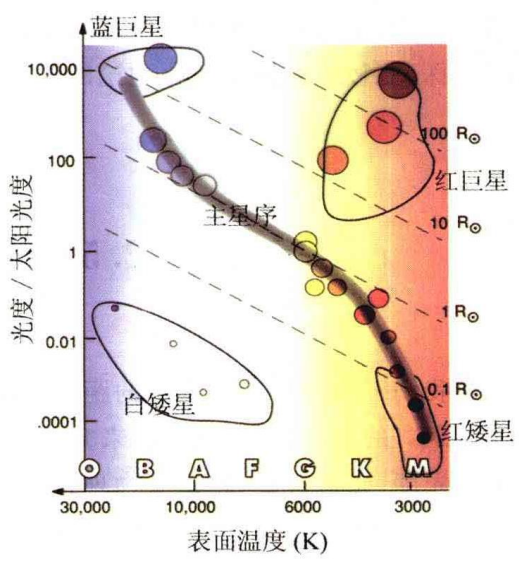## 一、基本天体物理量

### 1.星等

（1）【视星等】：m
$$\frac{E_1}{E_2}=100^{\frac{ {m_2}-{m_1} }5}$$

m1、m2表示2颗星的视星等，E1、E2表示2颗星的亮度（观测者在其位置观察该星的亮度，非实际亮度）

（2）【绝对星等】：M

$$\frac{E_{10} }{E} = \frac{r^2}{10^2}$$结合视星等定义公式可得：$$M-m=5-5\text{lg}r$$
m-M称为【距离模数】

（3）【光度】：L

$$\lg\frac{L}{L_{\odot} }=\frac{1}{2.5}(M_{\odot}-M)$$

1.某一时刻肉眼可见恒星约3000颗，全年一共肉眼可见恒星约6000颗

2.由于地球大气对光的吸收和散射，观测的星光会变暗变红，称为【大气消光】，因此实际观测中需要进行消光改正。除此之外，星际空间存在的大量气体和尘埃也会对光进行吸收和散射，称为【星际红化】和【星际消光】，同样需要进行相应修正

3.观测同一恒星使用不同的测量方法或观测不同的波段会得到不同的视星等，人眼对波长约为550nm的黄绿光最敏感，所观测的星等为【目视星等】mv；早期照相底片对波长约为430nm的蓝紫光最敏感，所测星等为【照相星等】mp；黄绿滤光片配合照相底片得到【仿视星等】mpv；望远镜终端的光电光度计测得的为【光电星等】;用对各个波段辐射灵敏度均相同的探测器测得的【辐射星等】;表征恒星在整个电磁波段辐射总量的【热星等】

### 2.温度

（1）【色指数】：C
$$C=m_{p}-m_{v}或C=m_{p}-m_{pv}$$
（2）【色温度】：T

$$T = \frac{7200}{C + 0.64}\mathbf（{K}）$$

（3）【有效温度】：Te

$$L=4\pi R^2\sigma T_e^4\quad\Rightarrow\quad T_e=\left(\frac{L}{4\pi R^2\sigma}\right)^{1/4}$$

R为恒星的半径，σ为斯特藩-玻尔兹曼常量

### 3.光谱型

（1）【基尔霍夫定律】

• 每一种化学元素在高温下都能产生辐射而发出独特的明线光谱；
• 在低温下每一种元素都可以吸收自己能够发出的这些辐射，从而使光谱中的明线变成暗线。

（2）【光谱型】

### 4.赫罗图（1）横轴表示光谱型（或温度、或色指数），纵轴表示光度（或绝对星等）

（2）三个明显的恒星聚集区：

• 【主星序】：人类观测到的90%以上的恒星都位于主星序上（包括太阳），称为主序星。主序星阶段是恒星一生中最稳定的阶段，恒星在这个阶段停留的时间占到整个寿命的90%以上。
• 【红巨星和红超巨星】：半径比太阳大几十倍到几百倍。例如肉眼可见的大角星(牧夫座α星)、毕宿五(金牛座α星)是红巨星，参宿四(猎户座α星)和心宿二(天蝎座α星)是红超巨星。
• 【白矮星】：半径仅为太阳的1/40~1/100，例如天狼星伴星，半径比地球还小。白矮星是超新星爆发的产物，是一部分恒星演化的最终归宿。

（3）图中一组平行的虚线为恒星等半径线，由恒星表面有效温度Te定义式与太阳的Te定义式两式相除并带入太阳相关数据可得：
$$\operatorname{lg}\frac{ {R} }{ R_{\odot} }=8.49-0.2M-2\operatorname{lg}T_{e}$$

• 其中M为恒星的绝对星等。这样,以绝对星等为纵坐标，以lgTe为横坐标的赫罗图上，恒星的等半径线是一组平行线。因而，如果知道一颗星在赫罗图上的位置，就知道了它的半径。
• 同时也表明，温度相同的情况下，光度越大，其半径也越大。即巨星的光度大、体积大，而矮星的光度小，体积小。